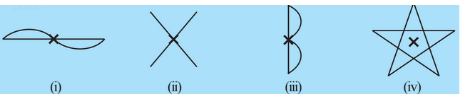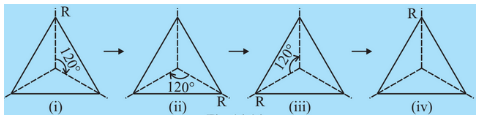## Filters

Sort by :
Clear All
Q

2.  Which of the following shapes (Fig 14.15) have rotational symmetry about the marked point.Among the above-given shapes, (i),(ii) and (iv) have rotational symmetry about the marked point.

1.(b) How many positions are there at which the triangle looks exactly the same, when rotated about its centre              by 120°?All the triangles look same when rotated by . Thus, there are 4 positions at which the triangle looks exactly the same when rotated about its centre by 120°.

1.(a) Can you now tell the order of the rotational symmetry for an equilateral triangle?An equilateral triangle has rotational symmetry at  angle. The order of the rotational symmetry for an equilateral triangle is 3.
Exams
Articles
Questions